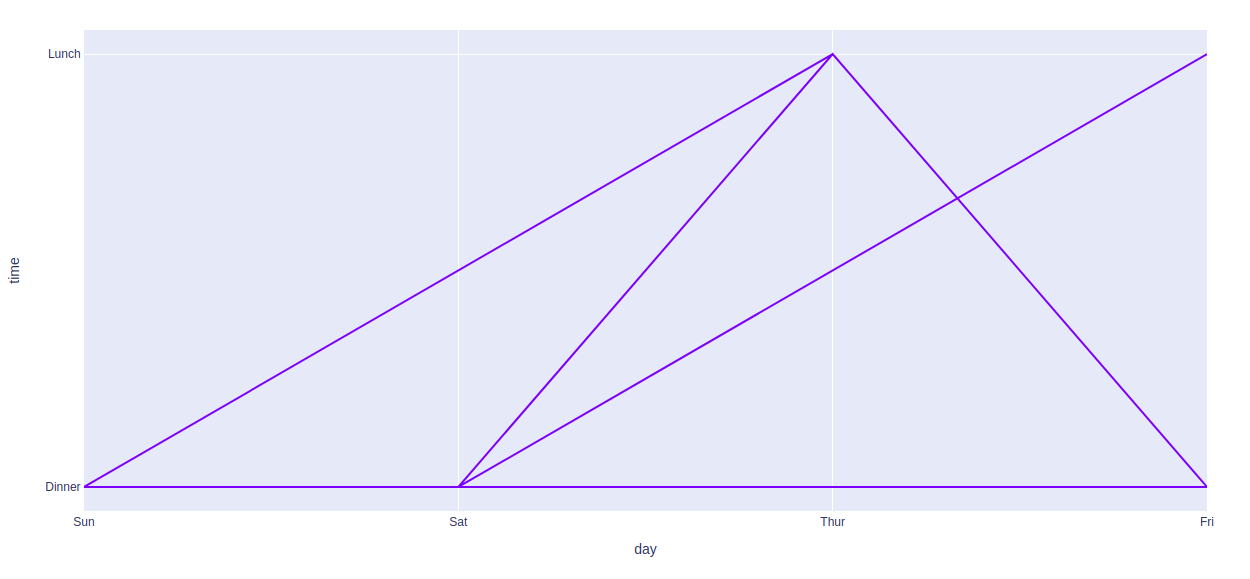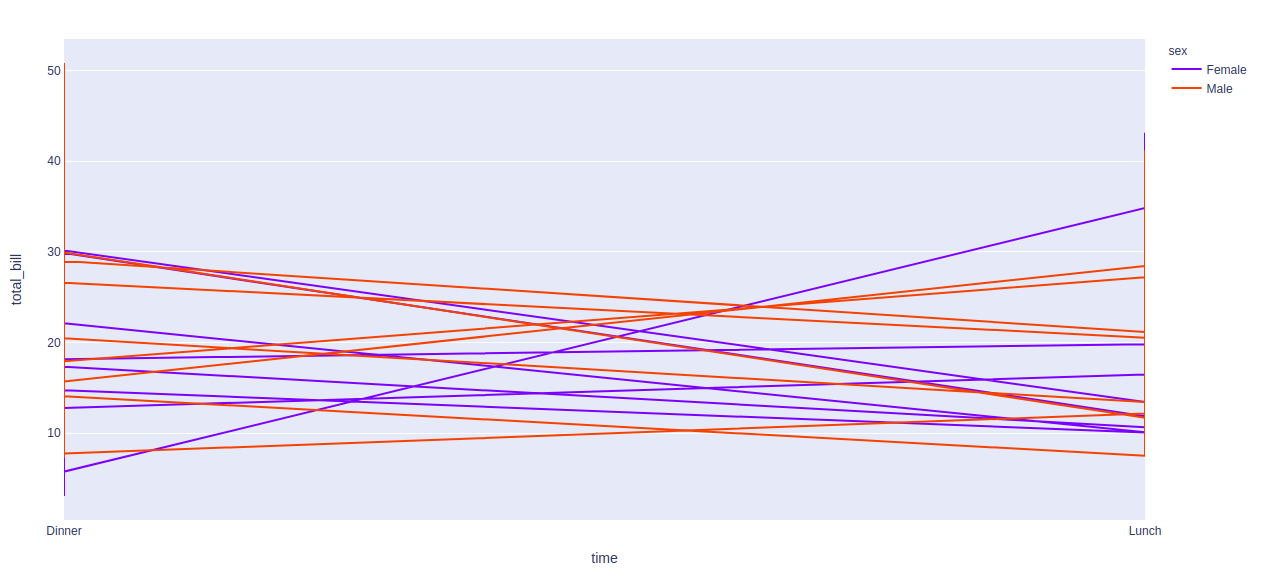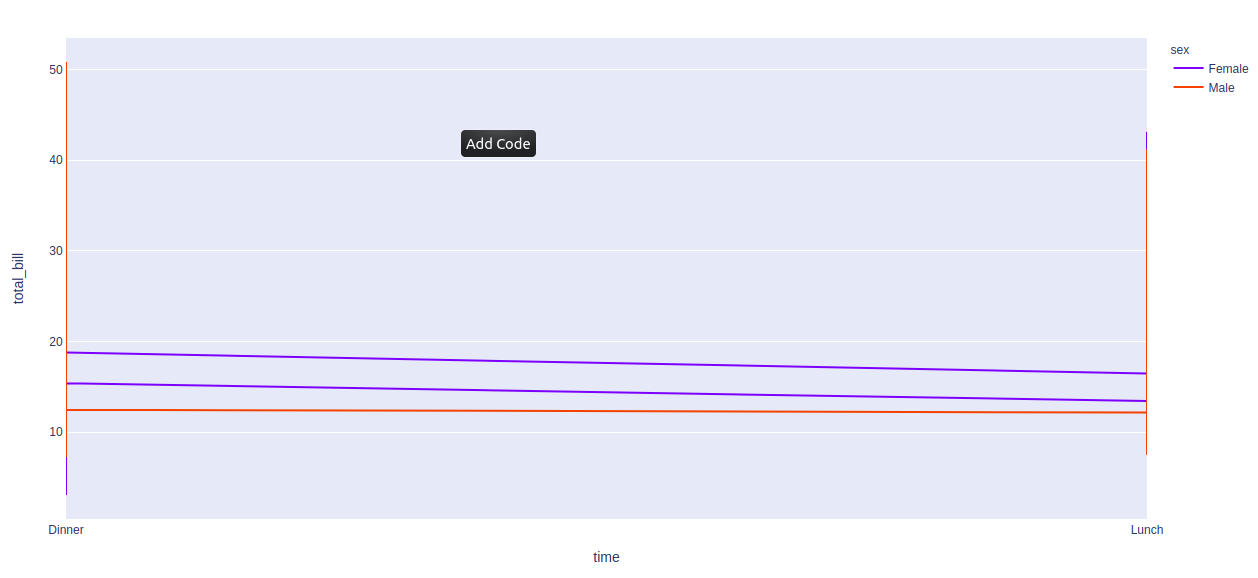# plotly.express.line() function in Python

• Last Updated : 20 Jul, 2020

Plotly library of Python can be very useful for data visualization and understanding the data simply and easily. Plotly graph objects are a high-level interface to plotly which are easy to use.

## plotly.express.line() function

This function is used to create a line plot. It can also be created using the pandas dataframe where each row of data_frame is represented as vertex of a polyline mark in 2D space.

Syntax: plotly.express.line(data_frame=None, x=None, y=None, line_group=None, color=None, line_dash=None, hover_name=None, hover_data=None, title=None, template=None, width=None, height=None)

Parameters:

data_frame: DataFrame or array-like or dict needs to be passed for column names.

x, y: This parameters is either a name of a column in data_frame, or a pandas Series or array_like object. Values from this column or array_like are used to position marks along the x and y axis in cartesian coordinates respectively.

color: This parameters assign color to marks.

line_group: This parameter is used to group rows of data_frame into lines.

line_dash: This parameter is used to assign dash-patterns to lines.

hover_name:  Values from this column or array_like appear in bold in the hover tooltip.

hover_data: This parameter is used to appear in the hover tooltip or tuples with a bool or formatting string as first element, and list-like data to appear in hover as second element Values from these columns appear as extra data in the hover tooltip.

Example 1:

## Python3

 `import` `plotly.express as px ` ` `  `df ``=` `px.data.tips() ` ` `  `plot ``=` `px.line(df, x ``=` `'day'``, y ``=` `'time'``) ` `plot.show()`

Output:Example 2: Using color argument

## Python3

 `import` `plotly.express as px ` ` `  `df ``=` `px.data.tips() ` ` `  `plot ``=` `px.line(df, x ``=` `'time'``,  ` `               ``y ``=` `'total_bill'``, ` `               ``color ``=` `'sex'``) ` `plot.show()`

Output:Example 3: Using the line_group argument

## Python3

 `import` `plotly.express as px ` ` `  `df ``=` `px.data.tips() ` ` `  `plot ``=` `px.line(df, x ``=` `'time'``, ` `               ``y ``=` `'total_bill'``, ` `               ``color ``=` `'sex'``,  ` `               ``line_group ``=` `'day'``) ` ` `  `plot.show()`

Output:My Personal Notes arrow_drop_up
Recommended Articles
Page :